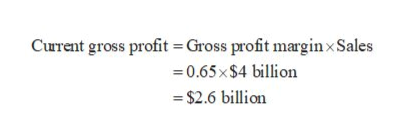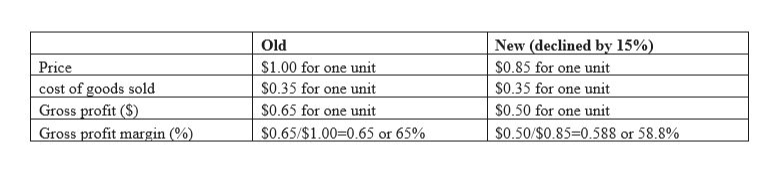Sample bartleby Q&A Solution
Company AF has been experiencing decline in the sales mainly because of intensive competition and weak economy. AF’s total sales were $4 billion last year. As an attempt to increase the sales, company is now considering to lower the prices on all items it sells. To make the idea of price reduction work successfully so that it increases the revenues, marketers must analyze by how much sales must go up. Assuming AF’s gross profit margin is 65 percent and cost of goods sold represents the only variable cost, by how much must sales increase to maintain the same gross profit margin in terms of absolute dollars if AF lowers prices by 15 percent? ## Expert Answer As the cost of goods sold represents the variable cost, the problem could be analyzed using the contribution analysis. As the price declines by 15%, the percentage of gross profit margin will decline as costs do not change with the reduction in the price. Now, to determine the amount of increase in sales required, we first compute the current gross profit.fullscreenExpand Although, the original prices are unknown, the scenario can be analyzed at a base price$1.00. As the gross profit margin is 65 percent, the unit gross profit would be equivalent to $0.65 for one unit and the unit cost of goods sold would be equivalent to$0.35 for one unit. Hence, the effect on unit gross profit and the gross margin % is seen when the price is declined by 15% to $0.85. Consider the table below showing old and new values after the decline in the price.fullscreenExpand As shown above, a reduction of 15% in price leads to a decline in the gross profit margin from 65 percent to 58.8 percent. Let us now compute the new sales level that is needed to break-even on this price decline, which should be achieved at the new gross profit margin to attain the actual gross profit of$2.6 billion.
The absolute raise in the sales to break even on a decrease of 15% in price is equivalent to $4,421,768,707 – %4,000,000,000 =$421,768,707. Thus, the sales should rise over this level for the decline in the price to be an efficient tactic.